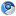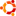[隐藏]

# 时间复杂度

## 1.时间频度

一个算法执行所耗费的时间，从理论上是不能算出来的，必须上机运行测试才能知道。但我们不可能也没有必要对每个算法都上机测试，只需知道哪个算法花费的时间多，哪个算法花费的时间少就可以了。并且一个算法花费的时间与算法中语句的执行次数成正比例，哪个算法中语句执行次数多，它花费时间就多。一个算法中的语句执行次数称为语句频度或时间频度。记为T(n)。

## 2.计算方法

1. 一般情况下，算法的基本操作重复执行的次数是模块n的某一个函数f（n），因此，算法的时间复杂度记做：T（n）=O（f（n））
分析：随着模块n的增大，算法执行的时间的增长率和f（n）的增长率成正比，所以f（n）越小，算法的时间复杂度越低，算法的效率越高。

2. 在计算时间复杂度的时候，先找出算法的基本操作，然后根据相应的各语句确定它的执行次数，再找出T（n）的同数量级（它的同数量级有以下：1，Log2n ，n ，nLog2n ，n的平方，n的三次方，2的n次方，n！），找出后，f（n）=该数量级，若T(n)/f(n)求极限可得到一常数c，则时间复杂度T（n）=O（f（n））

例：算法：

for（i=1;i<=n;++i）
{
for(j=1;j<=n;++j)
{
c[ i ][ j ]=0; //该步骤属于基本操作执行次数：n的平方 次
for(k=1;k<=n;++k)
c[ i ][ j ]+=a[ i ][ k ]*b[ k ][ j ]; //该步骤属于基本操作 执行次数：n的三次方 次
}
}

则有 T（n）= n的平方+n的三次方，根据上面括号里的同数量级，我们可以确定 n的三次方 为T（n）的同数量级
则有f（n）= n的三次方，然后根据T（n）/f（n）求极限可得到常数c
则该算法的 时间复杂度：T（n）=O（n的三次方）

## 3.分类

按数量级递增排列，常见的时间复杂度有：
常数阶O(1),对数阶O(log2n),线性阶O(n),
线性对数阶O(nlog2n),平方阶O(n2)，立方阶O(n3),…，
k次方阶O(nk), 指数阶O(2n) 。随着问题规模n的不断增大，上述时间复杂度不断增大，算法的执行效率越低。

# 空间复杂度

与时间复杂度类似，空间复杂度是指算法在计算机内执行时所需存储空间的度量。记作:
S(n)=O(f(n))
我们一般所讨论的是除正常占用内存开销外的辅助存储单元规模。

# 算法的时间复杂度（计算实例）

“大O记法”：在这种描述中使用的基本参数是 n，即问题实例的规模，把复杂性或运行时间表达为n的函数。这里的“O”表示量级 (order)，比如说“二分检索是 O(logn)的”,也就是说它需要“通过logn量级的步骤去检索一个规模为n的数组”记法 O ( f(n) )表示当 n增大时，运行时间至多将以正比于 f(n)的速度增长。

O(1)

Temp=i;i=j;j=temp;

O(n^2)

2.1. 交换i和j的内容

sum=0； （一次）
for(i=1;i<=n;i++) （n次）
for(j=1;j<=n;j++) （n^2次）
sum++； （n^2次）

2.2.

for (i=1;i<n;i++)
{
y=y+1; ①
for (j=0;j<=(2*n);j++)
x++; ②
}

f(n)=2n^2-n-1+(n-1)=2n^2-2

O(n)

2.3.
a=0;
b=1; ①
for (i=1;i<=n;i++) ②
{
s=a+b;　　　　③
b=a;　　　　　④
a=s;　　　　　⑤
}

T(n)=2+n+3(n-1)=4n-1=O(n).

O(log2n )

2.4.

i=1; ①
while (i<=n)
i=i*2; ②

T(n)=O(log2n )

O(n^3)

2.5.

for(i=0;i<n;i++)
{
for(j=0;j<i;j++)
{
for(k=0;k<j;k++)
x=x+2;
}
}

# 算法复杂度的渐近表示法

## 二  Ω记号

Ω记号与大O记号相反，他可以表示算法运行时间的下界。Ω（g（n））表示的函数集合的函数是所有阶数超过g(n)的函数。

## 三  Θ记号

Θ记号介于大O记号和Ω记号之间。他表示，存在正常数c1,c2,n0，当n>n0的时候，c1*g(n)≤f(n)≤c2*g(n)，则f(n)=Θ(g(n))。他表示所有阶数与g(n)相同的函数集合。

## 四  小o记号

f(n)=o(g(n))当且仅当f(n)=O(g(n))且f(n)≠Ω(g(n))。也就是说小o记号可以表示时间复杂度的上界，但是一定不等于下界。

## 五  例子

f(n)=Ω(n^2)或者f(n)=Ω(n)或者f(n)=Ω(1)

f(n)=Θ(n^2)

f(n) = o(n^3)或者f(n)=o(n^4)或者f(n)=o(n^5)或者……

# 常见排序算法时空复杂度

 排序法 最差时间分析 平均时间复杂度 稳定度 空间复杂度 冒泡排序 O(n2) O(n2) 稳定 O(1) 快速排序 O(n2) O(n*log2n) 不稳定 O(log2n)~O(n) 选择排序 O(n2) O(n2) 稳定 O(1) 二叉树排序 O(n2) O(n*log2n) 不一顶 O(n) 插入排序 O(n2) O(n2) 稳定 O(1) 堆排序 O(n*log2n) O(n*log2n) 不稳定 O(1) 希尔排序 O O 不稳定 O(1)

1.chuan说道：Chromium 45.0.2454.101Ubuntu x64

写得好啊,真厉害.明晰很多了,谢谢你2.Lvtu说道：

看不懂。。。[face_32]